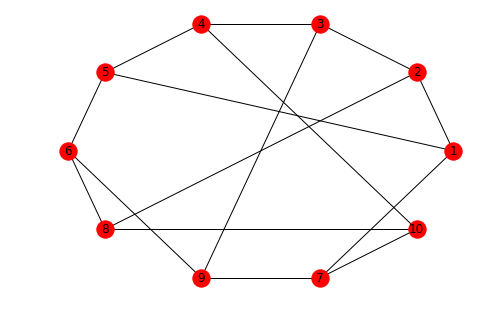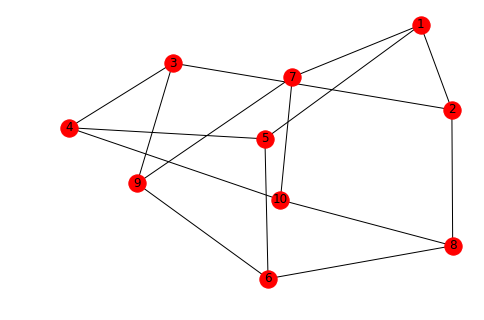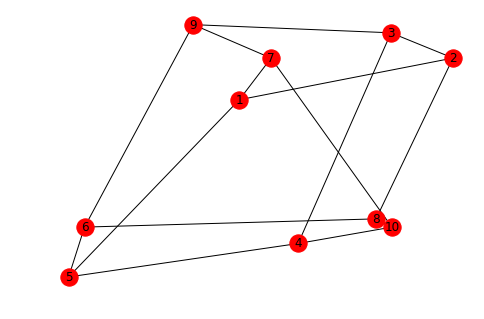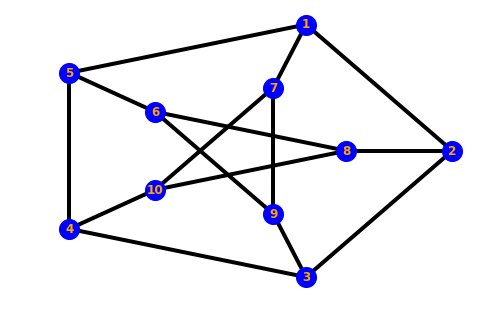# 1 Basics¶

In this warm-up notebook, we are going to assemble the following Petersen graph as a networkx.Graph. It might be useful to check the networkx documentation, and in particular this tutorial as we go along.In :
import numpy as np
import networkx as nx
import matplotlib.pyplot as plt


## 1.1. Dealing with edge lists¶

We can start our graph as an empty object, and introduce a single node via the following command:

In :
peterson_graph = nx.Graph() # Use nx.DiGraph if you want a directed graph.


We can also add the edge between nodes 1 and 2 by typing

In :
peterson_graph.add_edge(1,2)


Another possibility is to pass a list of edges to networkx:

In :
peterson_graph.add_edges_from([(2,3), (3,4)])


Now it is your turn. Add the remaining edges needed to construct the Peterson graph in the cell below.

In :
peterson_graph.add_edges_from([(4,5), (5,1), (5,6), (6,8), (6,9), (9,7), (8,10), (7,10), (7,1), (8,2), (9,3), (10,4)])


Verify that all the edges have been added by inspecting the output of the next cell.

In :
[e for e in peterson_graph.edges]

Out:
[(1, 2),
(1, 5),
(1, 7),
(2, 3),
(2, 8),
(3, 4),
(3, 9),
(4, 5),
(4, 10),
(5, 6),
(6, 8),
(6, 9),
(8, 10),
(9, 7),
(7, 10)]

Write the graph to file as in the edgelist format

In :
file_path = '../data/'
nx.write_edgelist(peterson_graph, file_path + 'peterson_graph.edgelist')


Load the edgelist file you have just created into a new networkx Graph

In :
peterson_graph_from_edgelist_file = nx.read_edgelist(file_path + 'peterson_graph.edgelist')


Verify that it is the same graph as before by running the cell below

In :
[e for e in peterson_graph_from_edgelist_file.edges]

Out:
[('1', '2'),
('1', '5'),
('1', '7'),
('2', '3'),
('2', '8'),
('5', '4'),
('5', '6'),
('7', '9'),
('7', '10'),
('3', '4'),
('3', '9'),
('8', '6'),
('8', '10'),
('4', '10'),
('9', '6')]

## 1.2. Dealing with adjacency matrices¶

We are going to create the same graph again, but this time we will use numpy to first assemble the graph's adjacency matrix. Then, we will create a networkx graph from this adjacency matrix.

Use the cell below to construct the adjacency matrix of the Petersen graph we have seen on the top of the notebook. Recall that an adjacency matrix $A$ is a $(\text{# nodes}) \times (\text{# nodes})$ matrix with $A_{ij} = 1$ if and only if node $i$ is connected to node $j$ and $A_{ij} = 0$ otherwise.

In :
adjacency = np.array([[0, 1, 0, 0, 1, 0, 1, 0, 0, 0],
[1, 0, 1, 0, 0, 0, 0, 1, 0, 0],
[0, 1, 0, 1, 0, 0, 0, 0, 1, 0],
[0, 0, 1, 0, 1, 0, 0, 0, 0, 1],
[1, 0, 0, 1, 0, 1, 0, 0, 0, 0],
[0, 0, 0, 0, 1, 0, 0, 1, 1, 0],
[1, 0, 0, 0, 0, 0, 0, 0, 1, 1],
[0, 1, 0, 0, 0, 1, 0, 0, 0, 1],
[0, 0, 1, 0, 0, 1, 1, 0, 0, 0],
[0, 0, 0, 1, 0, 0, 1, 1, 0, 0]
])


Now create a networkx graph from this matrix. It should be a one-line call.

In :
peterson_graph_from_adjacency = nx.from_numpy_array(adjacency)


At last, verify that you have the same graph as in Section 1.1 by examining the output of the following cell.

(Note that we had to increment the node labels by 1 because the adjacency matrix in indexed by default from 0.)

In :
[(1 + e, 1 + e) for e in peterson_graph_from_adjacency.edges]

Out:
[(1, 2),
(1, 5),
(1, 7),
(2, 3),
(2, 8),
(3, 4),
(3, 9),
(4, 5),
(4, 10),
(5, 6),
(6, 8),
(6, 9),
(7, 9),
(7, 10),
(8, 10)]

## 1.3 Graph visualization¶

The goal of this subsection is to show that the way we draw our graphs is in principle independent of the graph itself. In other words, the Euclidean distance in between points in the 2-dimensional visualizations below has nothing to do, a priori, with the properties of graph itsef. We will show that the same Petersen graph can have different, but equally correct representations on the 2d plane.

First, look into the networkx documentation about how to draw a circular layout for a given graph. Then, use the cell below to plot the Petersen graph using such a layout. Hint: set the parameter with_labels=True when drawing the graph, so you know which node is which.

In :
nx.draw_circular(peterson_graph, with_labels=True)
plt.show()

/home/michael/venv/amld2019_graph_workshop/lib/python3.7/site-packages/networkx/drawing/nx_pylab.py:611: MatplotlibDeprecationWarning: isinstance(..., numbers.Number)
if cb.is_numlike(alpha):Now, draw the Petersen graph using a spring layout. Does it look more like the first image we have seen of it? Try re-running the cell a couple of times.

In :
nx.draw_spring(peterson_graph, with_labels=True)
plt.show()You can use the cell below to experiment with other networkx (or otherwise) layouts.

In :
nx.draw_spectral(peterson_graph, with_labels=True)
plt.show()Finally, in case you are wondering whether we can reproduce the original drawing of the graph, run the cell below. Try to examine the documentation to discover how this was made possible.

In :
options = {
'node_color': 'blue',
'node_size': 400,
'width': 4,
'with_labels': True,
'font_color': 'orange',
'font_weight': 'bold'
}
shells = [[8, 7, 6, 10, 9], [2, 1, 5, 4, 3]]
nx.draw_shell(peterson_graph, nlist=shells, **options)